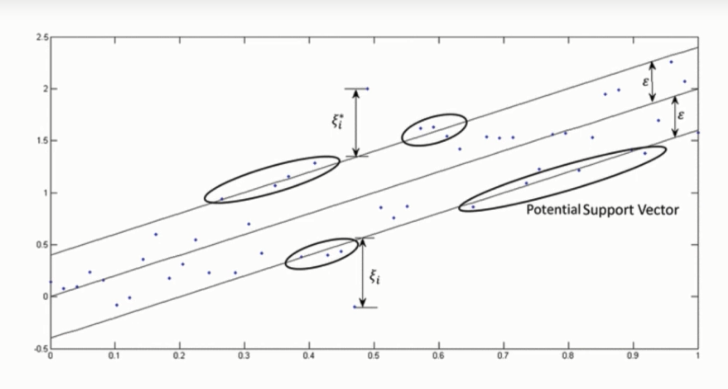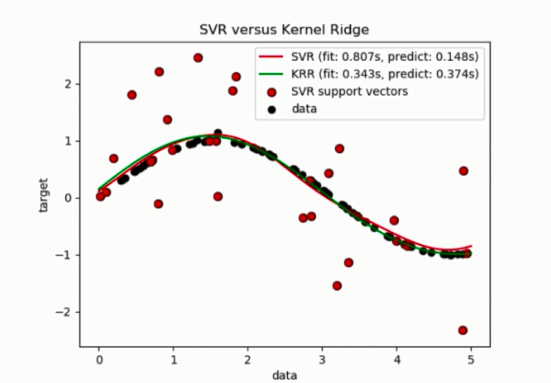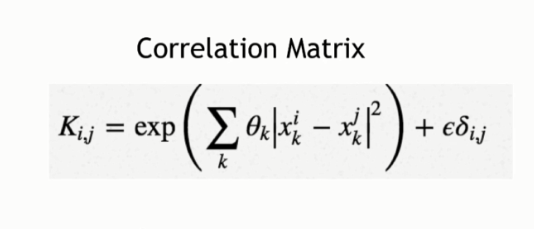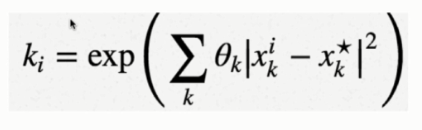Support Vector Regression | Machine Learning

Support Vector Regression: Support Vector Regression(SVR) is quite different than other Regression models. It uses Support Vector Machine(SVM, a classification algorithm) algorithm to predict a continuous variable. While other linear regression models try to minimize the error between the predicted and the actual value, Support Vector Regression tries to fit the best line within a predefined or threshold error value. What SVR does in this sense, it tries to classify all the prediction lines in two types, ones that pass through the error boundary( space separated by two parallel lines) and ones that donâ€™t. Those lines which do not pass the error boundary are not considered as the difference between the predicted value and the actual value has exceeded the error threshold, ðž®(epsilon). The lines that pass, are considered for a potential support vector to predict the value of an unknown. The following illustration will help you to grab this concept.To understand the above image, you first need to learn some important definitions.

1. Kernel: Kernel is a function that is used to map a lower dimensional data points into a higher dimensional data points. As SVR performs linear regression in a higher dimension, this function is crucial. There are many types of kernel such as Polynomial Kernel, Gaussian Kernel, Sigmoid Kernel etc.

2. Hyper Plane: In Support Vector Machine, a hyperplane is a line used to separate two data classes in a higher dimension than the actual dimension. In SVR, hyperplane is the line that is used to predict the continuous value.

3. Boundary Line: Two parallel lines drawn to the two sides of Support Vector with the error threshold value, ðž®(epsilon) are known as boundary line. This lines creates a margin between the data points.

4. Support Vector: The line from which the distance is minimum or least from two boundary data points.

From the above illustration, you clearly can find the idea. The boundary are trying to fit as much instances as possible without violating the margin. The width of the boundary is controlled by the error threshold ðž®(epsilon). In classification, the support vector X is used to define the hyperplane that separated the two different classes. Here, these vectors are used to perform linear regression.

How Does SVR Works?To perform an SVR, you must do the following steps:

• Collect a training set ð‰={ X,Y }

• Choose a Kernel and its parameters as well as any regularization needed

• Form the correlation matrix, K

• Train your machine, exactly or approximately, to get contraction coefficients, ={ i }

• Use these coefficients to create your estimator,  f(X , , x*) = y*

Lets do these steps one by one

Here we choose a Gaussian Kernel.

Now we come to the Correlation MatrixIn the equation above, we are evaluating our kernel for all pairs of points in our training set and adding the regulalizer resulting in the matrix.

The main part of the algorithm is, K = y

Here, yis the vector of values corresponding to your training set,

Kis the correlation matrix

And, is the set of unknowns we need to solve. Its value is obtained from the following equation,

= K-1y

Now as the parameter is known, we can form the estimator. We use all the coefficients we found during the optimization process and the kernel we started off with.

To estimate the unknown value, y*, for a test point x* we need the inner product of   and the correlation matrix K

y* = . K

Then we estimate the elements of coefficient matrix by the following:Overall, following all these steps, now our SVR model is ready to predict unknown values.